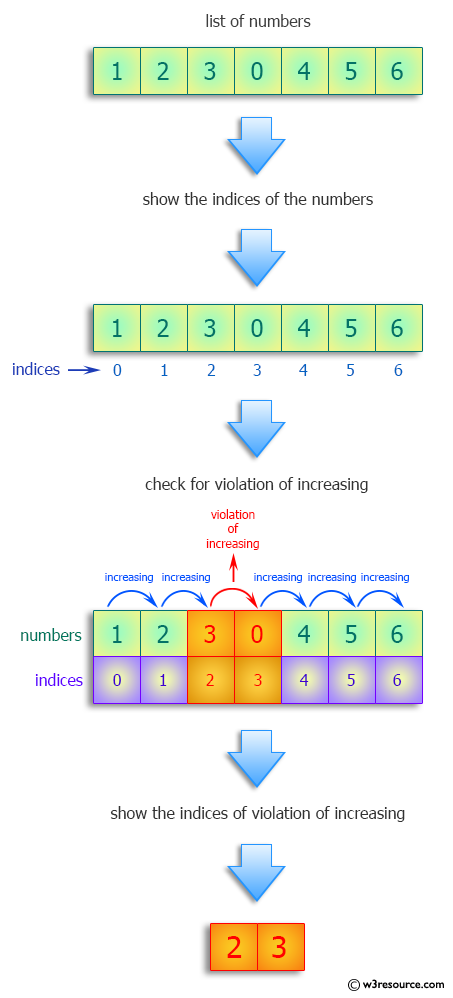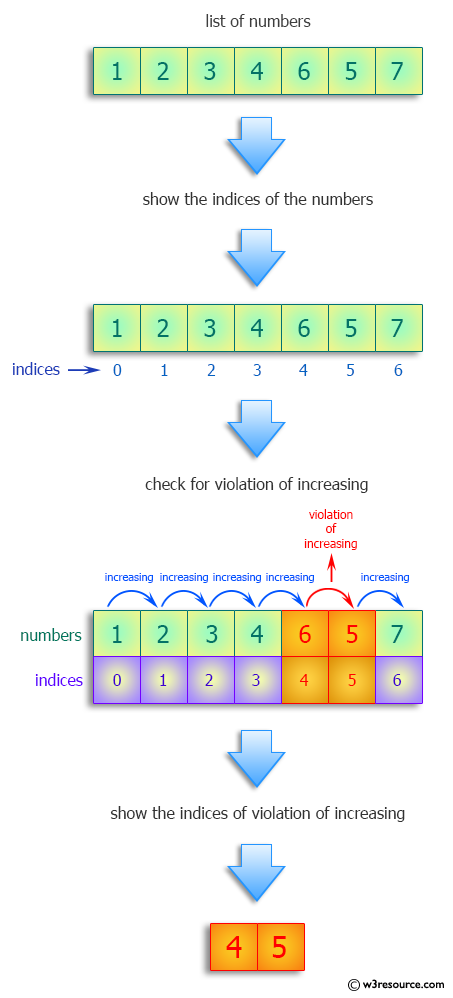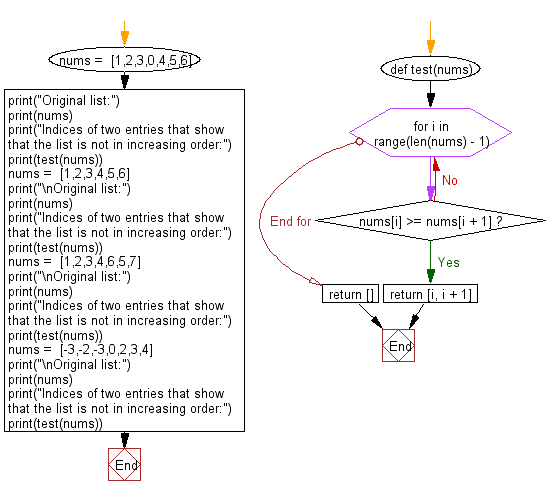﻿ Python: Find the indices of two entries that show that the list is not in increasing order - w3resource# Python: Find the indices of two entries that show that the list is not in increasing order

## Python Programming Puzzles: Exercise-48 with Solution

Write a Python program to find the indices of two entries that show that the list is not in increasing order. If there are no violations (they are increasing), return an empty list.

```Input:
[1, 2, 3, 0, 4, 5, 6]
Output:
[2, 3]

Input:
[1, 2, 3, 4, 5, 6]
Output:
[]

Input:
[1, 2, 3, 4, 6, 5, 7]
Output:
[4, 5]

Input:
[-3, -2, -3, 0, 2, 3, 4]
Output:
[1, 2]
```

Pictorial Presentation:Sample Solution:

Python Code:

``````#License: https://bit.ly/3oLErEI

def test(nums):
for i in range(len(nums) - 1):
if nums[i] >= nums[i + 1]:
return [i, i + 1]
return []

nums =  [1,2,3,0,4,5,6]
print("Original list:")
print(nums)
print("Indices of two entries that show that the list is not in increasing order:")
print(test(nums))
nums =  [1,2,3,4,5,6]
print("\nOriginal list:")
print(nums)
print("Indices of two entries that show that the list is not in increasing order:")
print(test(nums))
nums =  [1,2,3,4,6,5,7]
print("\nOriginal list:")
print(nums)
print("Indices of two entries that show that the list is not in increasing order:")
print(test(nums))
nums =  [-3,-2,-3,0,2,3,4]
print("\nOriginal list:")
print(nums)
print("Indices of two entries that show that the list is not in increasing order:")
print(test(nums))
``````

Sample Output:

```Original list:
[1, 2, 3, 0, 4, 5, 6]
Indices of two entries that show that the list is not in increasing order:
[2, 3]

Original list:
[1, 2, 3, 4, 5, 6]
Indices of two entries that show that the list is not in increasing order:
[]

Original list:
[1, 2, 3, 4, 6, 5, 7]
Indices of two entries that show that the list is not in increasing order:
[4, 5]

Original list:
[-3, -2, -3, 0, 2, 3, 4]
Indices of two entries that show that the list is not in increasing order:
[1, 2]
```

Flowchart:## Visualize Python code execution:

The following tool visualize what the computer is doing step-by-step as it executes the said program:

Python Code Editor :

Have another way to solve this solution? Contribute your code (and comments) through Disqus.

What is the difficulty level of this exercise?

Test your Programming skills with w3resource's quiz.

﻿

## Python: Tips of the Day

Clamps num within the inclusive range specified by the boundary values x and y:

Example:

```def tips_clamp_num(num,x,y):
return max(min(num, max(x, y)), min(x, y))
print(tips_clamp_num(2, 4, 6))
print(tips_clamp_num(1, -1, -6))
```

Output:

```4
-1
```